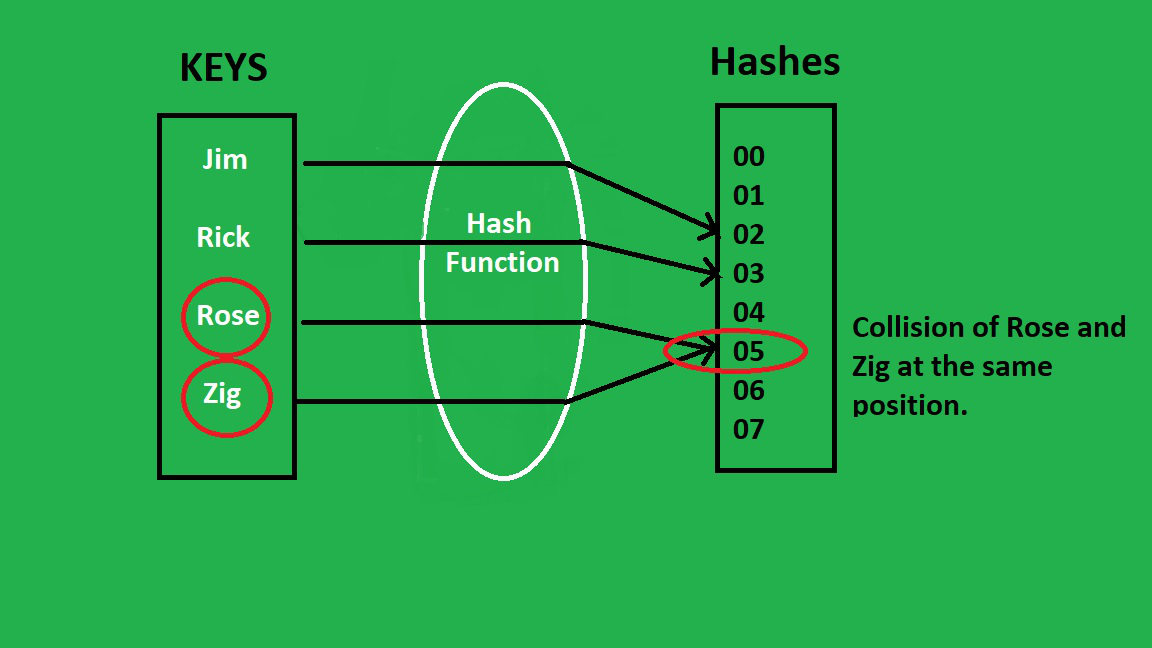Related Articles
Java Program to Implement Hash Tables Chaining with Doubly Linked Lists
• Difficulty Level : Expert
• Last Updated : 19 Jan, 2021

Hash Tables(similar to tables in general) provide a subset of the dynamic set operations. Usually, a set of keys are mapped with some values based on certain relations. However, there might be situations when different keys map to the same position provided by the Hash function, which leads to a collision.One of the ways to overcome this situation is Hash Table Chaining

The chaining approach to resolve collisions deals with it by going ahead and putting all the keys that map to a slot in that slot but representing them as a linked list.

Hash Table chaining in Java is possible with both, Singly Linked List and Doubly Linked List. Though the implementation is same, the only difference is that Doubly Linked List allows two-way traversal i.e., the node contains a pointer to the next as well as the previous node. Thus, the complexity of insertion and deletion at a known position reduces to O(1) as compared to the Singly Linked List (O(n)).

Example :

```In Singly Linked List : g -> e -> e -> k -> s
Here, each node contains a pointer to the next node only.

In Doubly Linked List : g <-> e <-> e <-> k <-> s
Each node contains pointer to the next as well as previous node.```

Approach :

1. Insertion: To insert, a key is simply hashed to get the position in the table (the list where this new key has to be inserted), and then insert the key at the head of the doubly linked list using the standard linked list procedures (given in the code).
2. Deletion: Just go through the list that the key maps to through the hash function and delete the key from that list using the standard linked list procedures (given in the code).

Below is the implementation of the above approach:

## Java

 `// Java implementation of Hashtable chaining``// using doubly linked list`` ` `import` `java.util.*;`` ` `class` `DoublyLinkedListNode {``    ``// Declaration of Nodes``    ``DoublyLinkedListNode next, prev;``    ``int` `data;`` ` `    ``// constructor``    ``DoublyLinkedListNode(``int` `data)``    ``{``        ``this``.data = data;``        ``next = ``null``;``        ``prev = ``null``;``    ``}``}``class` `HashTableChainingDoublyLinkedList {``    ``// Declaration of Hash Table``    ``DoublyLinkedListNode[] hashTable;`` ` `    ``// stores the size of HashTable``    ``int` `size;`` ` `    ``// Constructor``    ``HashTableChainingDoublyLinkedList(``int` `hashTableSize)``    ``{``        ``// Creating an empty Hash Table``        ``hashTable = ``new` `DoublyLinkedListNode[hashTableSize];``        ``size = ``0``;``    ``}`` ` `    ``// Function to check if hash table is empty``    ``public` `boolean` `isEmpty() { ``return` `size == ``0``; }`` ` `    ``// Function to clear/delete all elements from Hash table``    ``public` `void` `clear()``    ``{``        ``// Capacity of Hash Table``        ``int` `len = hashTable.length;`` ` `        ``// Creating new empty Hash Table``        ``// of same initial capacity``        ``hashTable = ``new` `DoublyLinkedListNode[len];``        ``size = ``0``;``    ``}`` ` `    ``// Function that returns size of Hash Table``    ``public` `int` `getSize() { ``return` `size; }`` ` `    ``// Function to insert a value/element``    ``public` `void` `insert(``int` `value)``    ``{``        ``size++;`` ` `        ``// gets the position/index where the value should be``        ``// stored``        ``int` `position = hash(value);`` ` `        ``// creates a node for storing value``        ``DoublyLinkedListNode node``            ``= ``new` `DoublyLinkedListNode(value);`` ` `        ``DoublyLinkedListNode start = hashTable[position];`` ` `        ``if` `(hashTable[position] == ``null``)``            ``hashTable[position] = node;``        ``else` `{``            ``node.next = start;``            ``start.prev = node;``            ``hashTable[position] = node;``        ``}``    ``}`` ` `    ``// Function to remove an element``    ``public` `void` `remove(``int` `value)``    ``{``        ``try` `{``            ``// gets the position where the value to``            ``// be deleted exists``            ``int` `position = hash(value);`` ` `            ``DoublyLinkedListNode start``                ``= hashTable[position];`` ` `            ``DoublyLinkedListNode end = start;`` ` `            ``// if value is found at start``            ``if` `(start.data == value) {``                ``size--;``                ``if` `(start.next == ``null``) {``                    ``// removing the value``                    ``hashTable[position] = ``null``;``                    ``return``;``                ``}`` ` `                ``start = start.next;``                ``start.prev = ``null``;``                ``hashTable[position] = start;`` ` `                ``return``;``            ``}`` ` `            ``// traversing the list``            ``// until the value is found``            ``while` `(end.next != ``null``                   ``&& end.next.data != value)``                ``end = end.next;`` ` `            ``// if reached at the end without finding the``            ``// value``            ``if` `(end.next == ``null``) {``                ``System.out.println(``"\nElement not found\n"``);``                ``return``;``            ``}`` ` `            ``size--;`` ` `            ``if` `(end.next.next == ``null``) {``                ``end.next = ``null``;``                ``return``;``            ``}`` ` `            ``end.next.next.prev = end;``            ``end.next = end.next.next;`` ` `            ``hashTable[position] = start;``        ``}``        ``catch` `(Exception e) {``            ``System.out.println(``"\nElement not found\n"``);``        ``}``    ``}`` ` `    ``// Definition of Hash function``    ``private` `int` `hash(Integer x)``    ``{``        ``int` `hashValue = x.hashCode();`` ` `        ``hashValue %= hashTable.length;`` ` `        ``if` `(hashValue < ``0``)``            ``hashValue += hashTable.length;`` ` `        ``return` `hashValue;``    ``}`` ` `    ``// Function to print hash table``    ``public` `void` `printHashTable()``    ``{``        ``System.out.println();``        ``for` `(``int` `i = ``0``; i < hashTable.length; i++) {``            ``System.out.print(``"At "` `+ i + ``":  "``);`` ` `            ``DoublyLinkedListNode start = hashTable[i];`` ` `            ``while` `(start != ``null``) {``                ``System.out.print(start.data + ``" "``);``                ``start = start.next;``            ``}`` ` `            ``System.out.println();``        ``}``    ``}`` ` `    ``// Driver Code``    ``public` `static` `void` `main(String[] args)``    ``{``        ``HashTableChainingDoublyLinkedList hashTab``            ``= ``new` `HashTableChainingDoublyLinkedList(``5``);`` ` `        ``hashTab.insert(``99``);``        ``hashTab.insert(``23``);``        ``hashTab.insert(``36``);``        ``hashTab.insert(``47``);``        ``hashTab.insert(``80``);`` ` `        ``hashTab.printHashTable();`` ` `        ``hashTab.insert(``92``);``        ``hashTab.insert(``49``);`` ` `        ``hashTab.printHashTable();`` ` `        ``hashTab.remove(``99``);`` ` `        ``hashTab.printHashTable();`` ` `        ``hashTab.clear();`` ` `        ``hashTab.printHashTable();`` ` `        ``System.out.println(hashTab.isEmpty());``    ``}``}`
Output
```At 0:  80
At 1:  36
At 2:  47
At 3:  23
At 4:  99

At 0:  80
At 1:  36
At 2:  92 47
At 3:  23
At 4:  49 99

At 0:  80
At 1:  36
At 2:  92 47
At 3:  23
At 4:  49

At 0:
At 1:
At 2:
At 3:
At 4:
true
```

Attention reader! Don’t stop learning now. Get hold of all the important Java Foundation and Collections concepts with the Fundamentals of Java and Java Collections Course at a student-friendly price and become industry ready. To complete your preparation from learning a language to DS Algo and many more,  please refer Complete Interview Preparation Course.

My Personal Notes arrow_drop_up